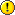# Difference between revisions of "Split text by symbol"

Split text by symbol (another string, also called delimiter or separator)

## Approaches

list of equivalent approaches to split text by symbol

## Example

Task: Split the position e.g. 25.040215, 121.512532 by the comma ( , ) to obtain the latitude (1st string) and longitude (2nd string).

Expected output:

• 25.040215
• 121.512532

### MySQL ApproachOnly one symbol was allowed. The following query will get the last element if there are more than two symbols.

1. to get the first string ( 25.040215 ): `SELECT TRIM(SUBSTRING_INDEX('25.040215, 121.512532' ,',', 1))`
2. to get the second string ( 121.512532 ): `SELECT TRIM(SUBSTRING_INDEX('25.040215, 121.512532' ,',', -1))`
```CREATE TABLE tbl(str varchar(50));
INSERT INTO tbl VALUES('25.040215, 121.512532');
INSERT INTO tbl VALUES('25.040215, 121.512532, 999.999'); /*bad data: 2 or more symbols*/
INSERT INTO tbl VALUES('123.456'); /*bad data: no symbol exists*/
SELECT TRIM(SUBSTRING_INDEX(str ,',', 1)), TRIM(SUBSTRING_INDEX(str ,',', -1)) FROM tbl WHERE str LIKE '%,%';
```

### Excel Approach

Comparison of approaches

Approach Output
Approach 1: Parse data Multiple parts will be returned if the content contains two or more separator characters.
Approach 2: SUBSTRING_INDEX Only two parts will be returned if the content contains two or more separator characters.

#### Approach 2: SUBSTRING_INDEX

A B C
1 25.040215, 121.512532
25.040215
121.512532

If the data set is perfect

1. separator character: ,
2. to get the first string ( 25.040215 ): B1 = `MID( A1, 1, FIND(",", A1)-1)`
3. to get the second string ( 121.512532 ): C1 = `MID( A1, FIND(",", A1)+1, LEN(A1))`

if the data set was mixed with empty values. Return empty if the value is empty.

1. to get the first string ( 25.040215 ): B1 = `IF(ISERROR(MID(A1, 1, FIND(",", A1)-1)), "", MID(A1, 1, FIND(",", A1)-1))`
2. to get the second string ( 121.512532 ): C1 = `IF(ISERROR(TRIM(MID(A1, FIND(",", A1)+1, LEN(A1)))), "", TRIM(MID(A1, FIND(",", A1)+1, LEN(A1)))`

online demo (allow to edit)

### PHP Approach

PHP script

```\$input_string = "25.040215, 121.512532";

\$list1 = explode(",", \$input_string);
\$trimmed_list1 = array_map('trim', \$list1);

\$list2 = preg_split("/[\s,]+/", \$input_string);

print_r(\$trimmed_list1);
print_r(\$list2);
```

output

```Array (
 => 25.040215
 => 121.512532
)
```